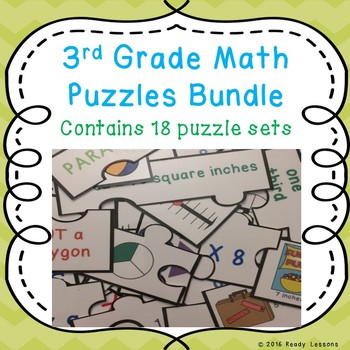# 3rd Grade Math Morning Work Review Game Puzzles BundleSubject
Resource Type
Format
Zip (65 MB|337 pages)
Standards
\$61.74
Bundle
List Price:
\$88.20
You Save:
\$26.46
\$61.74
Bundle
List Price:
\$88.20
You Save:
\$26.46

#### Products in this Bundle (18)

showing 1-5 of 18 products

### Description

Looking for a fun interactive teaching idea for 3rd grade math? Well look no further as this 3rd Grade Back to School Math Puzzles Bundle, aligned with grade 3 common core standards, will serve as exciting lesson plans for 3rd grade elementary school classrooms. This is a great resource to incorporate into your units as guided math center rotations, review game exercises, small group work, morning work, remediation, intervention or rti. It can also be used as a quiz, drill, test, or assessment tool to help determine student mastery of the learning targets. Whether a student is homeschooled or given this as a homework assignment, kids will also love working on these at home to improve their skills.

This math centers 3rd grade bundle includes 18 sets of puzzles to challenge your 3rd graders. Each puzzle set includes colorful jigsaw puzzles, answer key, and an optional station instruction page with an example. These come as pdf printable sheets that can be printed on card stock and laminated for long-term use. As a suggestion, store them in sealable gallon storage bags and place them in a tub or bin for students to use throughout the year for enrichment when they finish early. Another idea, for a project, is students can glue the completed puzzles into a notebook or journal as a reference sheet. They can also be glued on a poster for displaying on a bulletin board or as a wall anchor chart.

This 3rd grade math centers bundle covers many 3rd grade math standards in a fun way to keep students engaged. By matching the puzzle pieces to solve the problems, students can gain confidence in important and sometimes challenging skills. To challenge students further, you can time how quickly students can complete the math puzzles, and watch and see their time improve. Your 3rd graders will love to practice and learn to develop strategies about these math concepts.

I hope you download and enjoy these engaging hands-on manipulative back to school math activities bundle with your students! So set those worksheets aside and give our puzzles a try!

The puzzles in this bundle include:

Multiplication Puzzles: HERE

Division Puzzles: HERE

Multiplication and Division Word Problems Puzzles: HERE

Commutative Property of Multiplication Puzzles: HERE

Associative Property of Multiplication Puzzles: HERE

Distributive Property of Multiplication Puzzles: HERE

Rounding Numbers to the Nearest 10 and 100 Puzzles: HERE

Addition and Subtraction within 1000 Puzzles: HERE

Multiplication with Multiples of 10 Puzzles: HERE

Representing Fractions Puzzles: HERE

Comparing Fractions Puzzles: HERE

Telling Time to the Nearest Minute Puzzles: HERE

Elapsed Time Puzzles: HERE

Bar Graph and Pictograph Puzzles: HERE

Area and Perimeter Puzzles: HERE

Area and Perimeter Unit Squares Puzzles: HERE

Rectilinear Area and Perimeter Puzzles: HERE

Polygon Attribute Puzzles: HERE

Feedback:

Remember to leave product feedback to get TPT credits towards future purchases.

Also remember to follow my store by clicking the green star next to my logo for the latest product releases and freebies.

Total Pages
337 pages
Included
Teaching Duration
N/A
Report this Resource to TpT
Reported resources will be reviewed by our team. Report this resource to let us know if this resource violates TpT’s content guidelines.

### Standards

to see state-specific standards (only available in the US).
Understand that shapes in different categories (e.g., rhombuses, rectangles, and others) may share attributes (e.g., having four sides), and that the shared attributes can define a larger category (e.g., quadrilaterals). Recognize rhombuses, rectangles, and squares as examples of quadrilaterals, and draw examples of quadrilaterals that do not belong to any of these subcategories.
Explain equivalence of fractions in special cases, and compare fractions by reasoning about their size.
Understand a fraction as a number on the number line; represent fractions on a number line diagram.
Understand a fraction 1/𝘣 as the quantity formed by 1 part when a whole is partitioned into 𝘣 equal parts; understand a fraction 𝘢/𝑏 as the quantity formed by 𝘢 parts of size 1/𝘣.
Apply properties of operations as strategies to multiply and divide. Examples: If 6 × 4 = 24 is known, then 4 × 6 = 24 is also known. (Commutative property of multiplication.) 3 × 5 × 2 can be found by 3 × 5 = 15, then 15 × 2 = 30, or by 5 × 2 = 10, then 3 × 10 = 30. (Associative property of multiplication.) Knowing that 8 × 5 = 40 and 8 × 2 = 16, one can find 8 × 7 as 8 × (5 + 2) = (8 × 5) + (8 × 2) = 40 + 16 = 56. (Distributive property.)